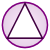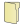Games
Problems
Go Pro!

# Triangles

Pro Problems > Math > Geometry > Triangles

# Featured Pro ProblemsAngle measures of a triangle, given information about the digits of the anglesIn a triangle the angles meet certain criteria. Can you determine what the angles are?Find the area of a triangle, given information about altitude and anglesAngles of three triangles are given in terms of x, y, and zAngles of an isosceles triangle - find x and y, and then the anglesTriangle, squares, area, and side length problemCan you find the missing angle in the described diagram?Find the value of y, given that the figure has the minimum possible perimeter# Full Directory Listing

Angle Bisector, Angle Measure, Angle Riddle, Angles in a Triangle, Arithmetic Angles, Forty-five and Thirty, Quadratic Triangle, Split Triangle, Three Triangles, Three Two-Digit Angle Measures, Triangle and Rectangle, Triangle in a Square, Triangle in a Square, Triangle Variables, Two Triangles with Angle Measures# Blogs on This SiteReviews and book lists - books we love!The site administrator fields questions from visitors.Like us on Facebook to get updates about new resources